xStructure factorEncyclopedia
In condensed matter physics
Condensed matter physics
Condensed matter physics deals with the physical properties of condensed phases of matter. These properties appear when a number of atoms at the supramolecular and macromolecular scale interact strongly and adhere to each other or are otherwise highly concentrated in a system. The most familiar...

and crystallography
Crystallography
Crystallography is the experimental science of the arrangement of atoms in solids. The word "crystallography" derives from the Greek words crystallon = cold drop / frozen drop, with its meaning extending to all solids with some degree of transparency, and grapho = write.Before the development of...

, the static structure factor (or structure factor for short) is a mathematical description of how a material scatters incident radiation. The structure factor is a particularly useful tool in the interpretation of interference patterns obtained in X-ray, electron
Electron diffraction
Electron diffraction refers to the wave nature of electrons. However, from a technical or practical point of view, it may be regarded as a technique used to study matter by firing electrons at a sample and observing the resulting interference pattern...

and neutron
Neutron diffraction
Neutron diffraction or elastic neutron scattering is the application of neutron scattering to the determination of the atomic and/or magnetic structure of a material: A sample to be examined is placed in a beam of thermal or cold neutrons to obtain a diffraction pattern that provides information of...

diffraction
Diffraction
Diffraction refers to various phenomena which occur when a wave encounters an obstacle. Italian scientist Francesco Maria Grimaldi coined the word "diffraction" and was the first to record accurate observations of the phenomenon in 1665...

experiments.

The static structure factor is measured without resolving the energy of scattered photons/electrons/neutrons. Energy-resolved measurements yield the dynamic structure factor
Dynamic structure factor
In condensed matter physics, the dynamic structure factor is a mathematical function that contains information about inter-particle correlations and their time evolution...

.

## Scattering from a crystal

A crystal
Crystal
A crystal or crystalline solid is a solid material whose constituent atoms, molecules, or ions are arranged in an orderly repeating pattern extending in all three spatial dimensions. The scientific study of crystals and crystal formation is known as crystallography...

is a periodic arrangement of atoms in a particular pattern. Each of the atoms may scatter incident radiation such as X-rays, electron
Electron
The electron is a subatomic particle with a negative elementary electric charge. It has no known components or substructure; in other words, it is generally thought to be an elementary particle. An electron has a mass that is approximately 1/1836 that of the proton...

s and neutron
Neutron
The neutron is a subatomic hadron particle which has the symbol or , no net electric charge and a mass slightly larger than that of a proton. With the exception of hydrogen, nuclei of atoms consist of protons and neutrons, which are therefore collectively referred to as nucleons. The number of...

s. Because of the periodic arrangement of the atoms, the interference of waves scattered from different atoms may cause a distinct pattern of constructive and destructive interference to form. This is the diffraction pattern caused by the crystal.

In the kinematical approximation for diffraction, the intensity of a diffracted beam is given by: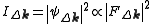where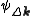is the wavefunction of a beam scattered a vector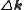, and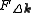is the so called structure factor which is given by: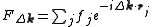Here,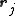is the position of an atom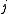in the unit cell, and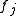is the scattering power of the atom, also called the atomic form factor
Atomic form factor
In physics, the atomic form factor, or atomic scattering factor, is a measure of the scattering amplitude of a wave by an isolated atom. The atomic form factor depends on the type of scattering, which in turn depends on the nature of the incident radiation, typically X-ray, electron or neutron...

. The sum is over all atoms in the unit cell. It can be shown that in the ideal case, diffraction only occurs if the scattering vectoris equal to a reciprocal lattice
Reciprocal lattice
In physics, the reciprocal lattice of a lattice is the lattice in which the Fourier transform of the spatial function of the original lattice is represented. This space is also known as momentum space or less commonly k-space, due to the relationship between the Pontryagin duals momentum and...

vector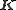.

The structure factor describes the way in which an incident beam is scattered by the atoms of a crystal unit cell, taking into account the different scattering power of the elements through the term. Since the atoms are spatially distributed in the unit cell, there will be a difference in phase when considering the scattered amplitude from two atoms. This phase shift is taken into account by the complex exponential term. The atomic form factor, or scattering power, of an element depends on the type of radiation considered. Because electrons interact with matter through different processes than, for example, X-rays, the atomic form factors for the two cases are not the same.

## Structure factors for specific lattice types

To compute structure factors for a specific lattice, compute the sum above over the atoms in the unit cell. Since crystals are often described in terms of their Miller indices
Miller index
Miller indices form a notation system in crystallography for planes and directions in crystal lattices.In particular, a family of lattice planes is determined by three integers h, k, and ℓ, the Miller indices. They are written , and each index denotes a plane orthogonal to a direction in the...

, it is useful to examine a specific structure factor in terms of these.

Body-centered cubic (BCC)

As a convention, the body-centered cubic system is described in terms of a simple cubic lattice with primitive vectors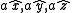, with a basis consisting of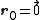and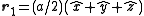. The corresponding reciprocal lattice is also simple cubic with side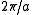.

In a monoatomic crystal, all the form factors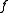are the same. The intensity of a diffracted beam scattered with a vector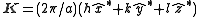by a crystal plane with Miller indices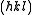is then given by: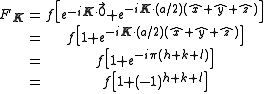We then arrive at the following result for the structure factor for scattering from a plane: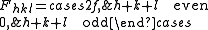This result tells us that for a reflection to appear in a diffraction experiment involving a body-centered crystal, the sum of the Miller indices of the scattering plane must be even. If the sum of the Miller indices is odd, the intensity of the diffracted beam is reduced to zero due to destructive interference. This zero intensity for a group of diffracted beams is called a systematic absence. Since atomic form factors fall off with increasing diffraction angle corresponding to higher Miller indices, the most intense diffraction peak from a material with a BCC structure is typically the (110). The (110) plane is the most densely packed of BCC crystal structures and is therefore the lowest energy surface for a thin film to grow. Films of BCC materials like iron
Iron
Iron is a chemical element with the symbol Fe and atomic number 26. It is a metal in the first transition series. It is the most common element forming the planet Earth as a whole, forming much of Earth's outer and inner core. It is the fourth most common element in the Earth's crust...

and tungsten
Tungsten
Tungsten , also known as wolfram , is a chemical element with the chemical symbol W and atomic number 74.A hard, rare metal under standard conditions when uncombined, tungsten is found naturally on Earth only in chemical compounds. It was identified as a new element in 1781, and first isolated as...

therefore grow in a characteristic (110) orientation.

Face-centered cubic (FCC)

In the case of a monoatomic FCC crystal, the atoms in the basis are at the originwith indices (0,0,0) and at the three face centers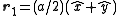,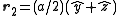,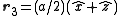with indices given by (1/2,1/2,0), (0,1/2,1/2), (1/2,0,1/2). An argument similar to the one above gives the expression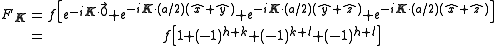with the result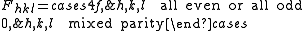The most intense diffraction peak from a material that crystallizes in the FCC structure is typically the (111). Films of FCC materials like gold
Gold
Gold is a chemical element with the symbol Au and an atomic number of 79. Gold is a dense, soft, shiny, malleable and ductile metal. Pure gold has a bright yellow color and luster traditionally considered attractive, which it maintains without oxidizing in air or water. Chemically, gold is a...

tend to grow in a (111) orientation with a triangular surface symmetry.

Diamond Crystal Structure

The Diamond cubic
Diamond cubic
The diamond cubic crystal structure is a repeating pattern of 8 atoms that certain materials may adopt as they solidify. While the first known example was diamond, other elements in group IV also adopt this structure, including tin, the semiconductors silicon and germanium, and silicon/germanium...

crystal structure occurs in diamond
Diamond
In mineralogy, diamond is an allotrope of carbon, where the carbon atoms are arranged in a variation of the face-centered cubic crystal structure called a diamond lattice. Diamond is less stable than graphite, but the conversion rate from diamond to graphite is negligible at ambient conditions...

(carbon
Carbon
Carbon is the chemical element with symbol C and atomic number 6. As a member of group 14 on the periodic table, it is nonmetallic and tetravalent—making four electrons available to form covalent chemical bonds...

), most semiconductors and tin
Tin
Tin is a chemical element with the symbol Sn and atomic number 50. It is a main group metal in group 14 of the periodic table. Tin shows chemical similarity to both neighboring group 14 elements, germanium and lead and has two possible oxidation states, +2 and the slightly more stable +4...

. The basis cell contains 8 atoms located at cell positions: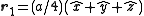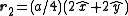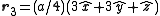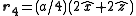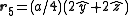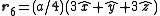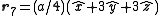The Structure factor then takes on a form like this: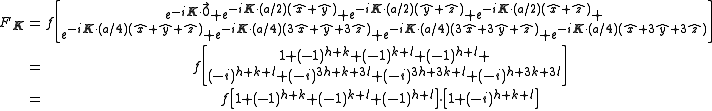with the result
• for mixed values (odds and even values combined) of h, k, and l, F2 will be 0

• if the values are unmixed and...
• h+k+l is odd then F=4f(1+i) or 4f(1-i), FF*=32f2
• h+k+l is even and exactly divisible by 4 (satisfies h+k+l=4n) then F = 8f
• h+k+l is even but not exactly divisible by 4(doesn't satisfy h+k+l=4n) then F = 0

## Polymers

In polymeric systems, the static structure factor describes the intensity of scattered light as a function of angle of scattering. It is defined by the following equation :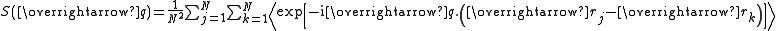In this definition, a polymer chain is assumed to be made up of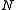beads. The other terms are defined as: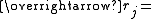Position vector of bead j of the polymer chain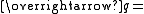Scattering vector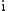denotes the imaginary unit
Imaginary unit
In mathematics, the imaginary unit allows the real number system ℝ to be extended to the complex number system ℂ, which in turn provides at least one root for every polynomial . The imaginary unit is denoted by , , or the Greek...

The average is taken over all possible pairs j,k that belong to the same chain.